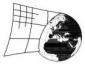TLoELaG 2016/01/18

3 پاسخ [آخرین پست]
آفلاین
عضو شده: 11/04/2013

Hi!
I had a problem with solving today's puzzle. Then, I've realised, I misunderstood the rules because of this sentence:

"The number in a circle is the sum of the odd digits along its arrow."

For me, the "SUM" of odd digits means: if there are 1 and 3 on the arrow in a central square, so in the circle must be 4 (1+3). Thinking this way, I realised, that's the only possibility for this arrow and then I came soon to a wrong situation in a right down corner square. It came to me, the grid is broken. But when I've opened the link to the Author's site, I read, that in the circle should be a NUMBER (an AMOUNT; HOW MUCH) odd digits on the arrow. So, I think, the description of the rules is misleading.آفلاین
عضو شده: 10/12/2008
Wrong Rules

k3isim wrote:
Hi!
I had a problem with solving today's puzzle. Then, I've realised, I misunderstood the rules because of this sentence:

"The number in a circle is the sum of the odd digits along its arrow."

For me, the "SUM" of odd digits means: if there are 1 and 3 on the arrow in a central square, so in the circle must be 4 (1+3). Thinking this way, I realised, that's the only possibility for this arrow and then I came soon to a wrong situation in a right down corner square. It came to me, the grid is broken. But when I've opened the link to the Author's site, I read, that in the circle should be a NUMBER (an AMOUNT; HOW MUCH) odd digits on the arrow. So, I think, the description of the rules is misleading.

I'm so sorry, but I have misunderstood the Rules, and put it here in this wrong form.
This game will be canceled and won't be taken into monthly standings.

Sorry for that.
Kodyn

آفلاین
عضو شده: 01/29/2013
Same problem too!

Oh! Yes, I did the exact same thing: started with 4 in the middle circle, and quickly got into trouble in the low right nonet.

So it should be: the number in the circle is how many odd numbers there are on the arrow?

آفلاین
عضو شده: 01/14/2009